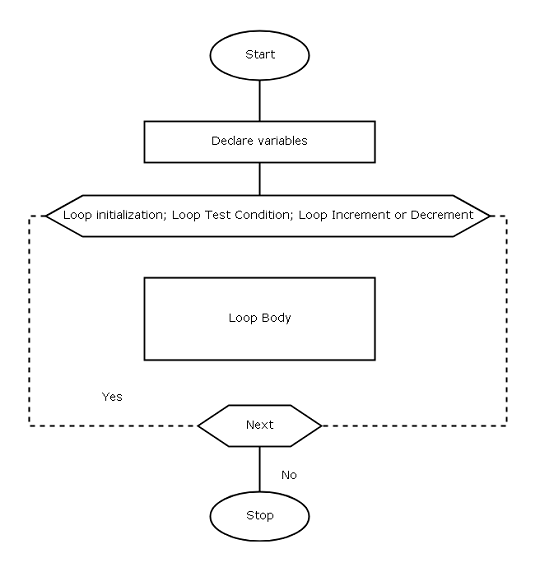## C Programming Tutorial – for Loop

The ‘for’ loop is a simpler version of the while loop that we learned in the previous lesson. It has the same components as while loop.

The for loop is more popular because all conditions for loop is declared in one place.

Before the for loop starts.

1. Loop variable – It is used for initializing the loop, test condition for loop and increment or decrement the loop.
2. Loop test condition– decide whether to execute the loop body or not.
3. Loop body – contains statements required for performing a task inside the loop.

Logically ‘while’ loop and ‘for’ loop is same but the ‘for’ loop is lot more simple than the ‘while’ loop. This is because you can initialize, put test conditions and increment or decrement the ‘for’ loop in a single line.

``````for( initialize counter; test condition; increment or decrement counter)

{

Statements;

}``````

#### Flowchart Symbol for ‘for’ loop

The following symbol is used when we draw a flowchart for a C program that make use of a ‘for’ loop.Diagram – For Loop

#### Example Program

In this example program, we are going to a number over and over for 10 times and print the result. This program uses a single ‘for’ loop.

``````/* C Program to add a number 10 times */

#include <stdio.h>

int main ()

{

int number, total, i;

number = 2;

total = 0;

for (i=0; i<10; i++)

{

total = total + number;

}

printf (“\n\n\n\n”);

printf (“\t\t\tThe total is %d”, total);

getch ();

return 0;

}``````

#### OutputOutput-for-loop

#### Example Explained

The program has a three variables – loop variable ‘i’, number and total and it uses one ‘for’ loop to repeatedly add the number to sum until the loop terminates.

First the variables number and total are initialized.

``````total = 0;

number = 2;``````

The loop variable ‘i’ is used inside the ‘for’ loop to initialize the loop, define the test condition and increment the loo.

``for (i =0; i<10; i++)``

Since the loop starts at i =0, it will run for 10 iteration because the 10th iteration it will terminate. At each iteration, the loop will check the test condition against the current value of ‘i’.

When the condition for loop is ‘true’, the loop body will execute and add value of variable number to total repeatedly.

``total = total + number;``

The loop add the number 10 times and when i = 10, it terminates and prints the value of total as output.

``printf (“\t\t\t\t The total is %d”, total);``

The program ends.

### Bibliography

Balagurusamy, E. 2000. Programming in ANSI C. Tata McGraw-Hill Education,.

Kanetkar, Yashavant. 20 November 2002. Let us C. Bpb Publications.